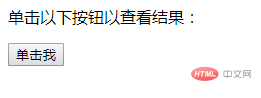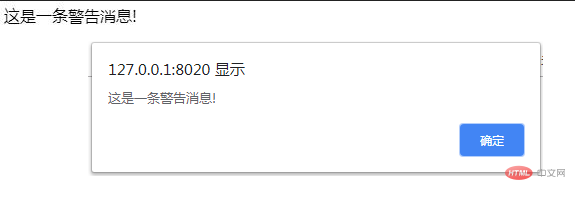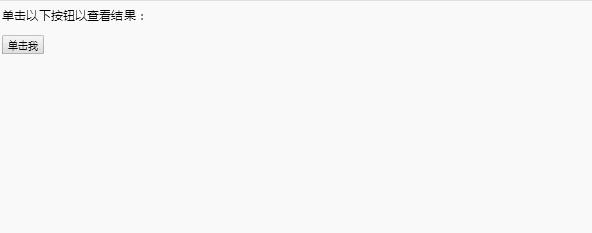ES 6 对话框

javascript支持三种重要的对话框类型。这些对话框可用于发出警报，或对任何输入进行确认，或从用户那里获得某种输入。这里我们将逐一讨论每个对话框。

```<html>
<meta charset="UTF-8">
<script type = "text/javascript">
function Warn() {
document.write ("这是一条警告消息!");
}
</script>

<body>
<p>单击以下按钮以查看结果： </p>
<form>
<input type = "button" value = "单击我" onclick = "Warn();" />
</form>
</body>
</html>``````<html>
<meta charset="UTF-8">
<script type = "text/javascript">
function getConfirmation(){
var retVal = confirm("是否继续？");

if( retVal == true ){
return true;
} else {
return false;
}
}
</script>

<body>
<p>单击以下按钮以查看结果： </p>
<form>
<input type = "button" value = "单击我" onclick = "getConfirmation();" />
</form>
</body>
</html>``````<html>
<meta charset="UTF-8">
<script type = "text/javascript">
function getValue(){
var retVal = prompt("输入你的姓名 : ", "你的名字在这里");
document.write("你已进入: " + retVal);
}
</script>

<body>
<p>单击以下按钮以查看结果：</p>
<form>
<input type = "button" value = "单击我" onclick = "getValue();" />
</form>
</body>
</html>```